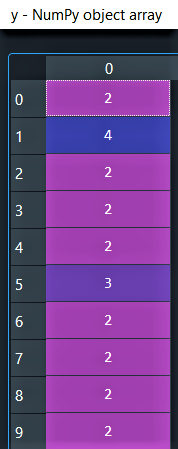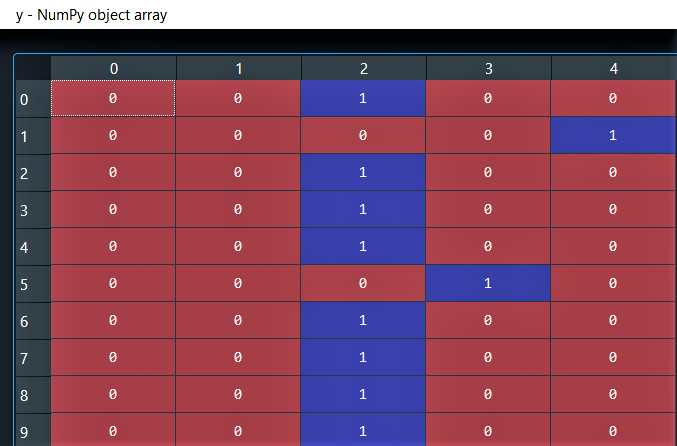# Solve ValueError: Error when checking target in Keras

This article will help you solve ValueError: Error when checking target in Keras which we usually encounter while working with neural networks in Deep Learning. You will learn how to resolve this error quickly in few steps but first let us understand what causes this error in our program.

## ValueError: Error when checking target in Keras

“target” indicates that the problem is present at the output of our model. This error generally occurs when the dimension of the output specified to the model is not equal to the dimension of the output dataset given to the model. To get a better understanding let us look at an example.

### Example :

Consider the code (in Python) given below :

```import pandas as pd
import numpy as np

# example_dataset is any pandas dataframe where suppose the last column is the target variable.
X = example_dataset.iloc[:, :-1].values
y = example_dataset.iloc[:, -1].values.reshape(-1, 1)```

Here, ‘X’ is my set of independent variables and ‘y’ is the target variable which is a categorical data.

```print(f'Number of rows & columns in X : {np.shape(X)}')
print(f'Number of rows & columns in y : {np.shape(y)}')
print(f'Number of categories in y : {len(np.unique(y))}')
print(f'Categories in y : {np.unique(y)}')```

Output :

```Number of rows & columns in X : (620, 28)
Number of rows & columns in y : (620, 1)
Number of categories in y : 5
Categories in y : [0 1 2 3 4]```

As we can see the target variable ‘y’ with dimensions (620, 1) has 5 categories in it labeled as [0, 1, 2, 3, 4]. Now let us try to fit an Artificial Neural network (ANN) on this dataset.

```import keras
from keras.models import Sequential
from keras.layers import Dense

# Initialising the ANN
model = Sequential()

# Adding the input layer and the first hidden layer of the ANN
model.add(Dense(output_dim = 17, init = 'uniform', activation = 'relu', input_dim = 28))

# Adding the output layer with output_dim = 5 as 'y' has 5 categories in it
model.add(Dense(output_dim = 5, init = 'uniform', activation = 'softmax'))

# Compiling the ANN
model.compile(optimizer = 'adam', loss = 'categorical_crossentropy', metrics = ['accuracy'])

# Fitting the ANN to the Training set
model.fit(X, y, batch_size = 32, nb_epoch = 25)```

The above code results in the following error :

```ValueError: Error when checking target: expected dense_28 to have shape (5,)
but got array with shape (1,)```

This error occurs because our target variable ‘y’ has a single column whereas we specified the number of columns in the target variable as 5 while adding the output layer of the ANN.  The model can compare its prediction with the correct answer only if they have the same dimension.

### How to resolve :

To resolve the above error, we have to make the dimension specified in the output layer equal to the dimension of the target variable i.e. we have to make the number of columns of our target variable ‘y’ equal to the value specified in the output_dim parameter of the output layer in the ANN. In order to do so, we have to perform OneHotEncoding on the target variable where we will assign one column to each category of the target variable ‘y’.

### Code | OneHotEncoding :

We will perform OneHotEncoding using ColumnTransformer and OneHotEncoder from the scikit-learn library.

```from sklearn.compose import ColumnTransformer
from sklearn.preprocessing import OneHotEncoder
ct = ColumnTransformer(transformers = [('encoder', OneHotEncoder(), )], remainder = 'passthrough')
y = ct.fit_transform(y).toarray()

print(f'Number of rows & columns in y : {np.shape(y)}')```

Output :

`Number of rows & columns in y : (620, 5)`

First 10 elements of ‘y’ before OneHotEncoding :First 10 elements of ‘y’ after OneHotEncoding :Now when we try to fit our model on the dataset, the ValueError: Error when checking target does not occur as we made the dimension of the target variable equal to the dimension that the model expected the target to have.